Higher-Dimensional Simplexes

The shadow image of an Egyptian pyramid is completely determined by the shadow of its apex. At any instant, the shadow of an edge along the base remains fixed, and the image of an edge leading from a base point to the apex will be a line joining the base point to the image of the apex. This fact is true for a pyramid with any shape base. For a triangular pyramid, as soon as we know the location of the shadows of the four vertices, we can draw the complete shadow of the transparent object--just connect aII possible pairs of vertices. Thus we can complete the shadow without ever having to construct the object.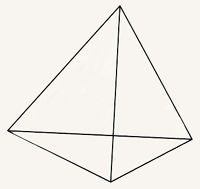A shadow of a triangular pyramid.

The triangle and the triangular pyramid have higher-dimensional analogues, known as simplexes. Three points in a plane, not lying in a line, determine a triangle, also called a two-simplex. Four points in space, not lying in a plane, determine a triangular pyramid, also called a three-simplex or tetrahedron. The n-simplex is the smallest figure that contains n + 1 given points in n-dimensional space and that does not lie in a space of lower dimension. If we analyze the numbers of edges, triangles, and other simplexes in an n-simplex, we find a pattern that occurs over and over again in algebra and in the study of probability. The reappearance of such patterns is one of the most beautiful aspects of mathematics.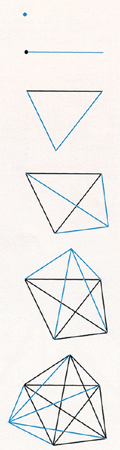The simplexes in the first six dimensions.

As we follow the procedure for drawing simplexes, we can count the number of edges. We start with a point. We then choose a distinct point and draw the 1 edge connecting it to the point we already had. We choose a new point, not on the line of the first 2 points, and connect it to the previous 2 points to get 2 more edges for a total of 3. The next step is to choose a new point not lying on any of the 3 lines determined by the edges already constructed, and we then connect this new point to the previous 3 points to get 3 new edges for a total of 6.

We repeat this process to draw the image of the simplest figure determined by 5 points, the four-simplex. We first choose a point not on any of the 6 lines containing previously constructed edges and then connect this point to the previous 4 points to obtain 4 new edges for a total of 10. We can imagine that the fifth point actually extends into a fourth dimension, and that we are observing the shadows of the segments connecting it to the 4 points of the three-simplex.

If we arrange the results in a table, a pattern emerges.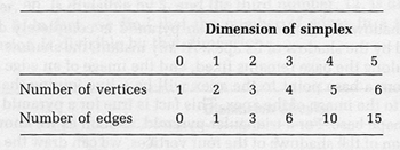The number of edges at any stage is the sum of all the numbers less than the number of that stage, so the number of edges formed by 6 points will be 1 + 2 + 3 + 4 + 5 = 15. This can easily be seen from our procedure since each new point is connected to all the previous points.

The study of combinations provides a different way of finding the number of edges of a simplex. Since each vertex is to be connected to every other vertex, the number of edges will be equal to the number of pairs of vertices, that is, the number of combinations of a certain number of vertices taken two at a time. If we have n + 1 vertices, then there are n + 1 choices for the first element of the pair and n remaining vertices for the second element. Multiplying these numbers gives (n + 1) times n, and since this counts each edge twice, the total number of edges is n(n + 1)/2.

Spatial perception tests are fond of asking students to extract a simple figure from a complicated one, and counting the edges is one of the most basic tasks. Next in difficulty would be counting the number of distinct triangles at each stage. The three-dimensional simplex has 4 triangles. The four-dimensional simplex has these 4 plus 6 new triangles formed by attaching the edges of the three-simplex to the new vertex, yielding a total of 10 triangles on the four-simplex. We can then extend the previous table.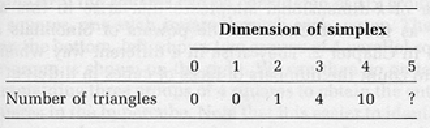At each stage, the number of triangles formed by a given number of points is the sum of the previous number of triangles and the previous number of edges. Thus the number of triangles in a five-dimensional simplex determined by 6 points is 10 + 10 = 20. In general, the number of triangles formed by n points will be (n - 1)(n - 2)/6. We have as many triangles as there are distinct triples of vertices, so we have the combinations of a certain number of objects taken three at a time. In general the number of k-dimensional simplexes in an n-dimensional simplex is the number of combinations of n + 1 elements taken k + 1 at a time. The formula for this number is:

C(n + 1, k + 1) = (n + 1)!/[(k + 1)!(n - k)!]

where n! stands for the product of the integers from 1 to n. The number of k-dimensional simplexes that arise in this analysis appear as the coefficients in the powers of binomials already treated in Chapter 2. They arise in a different way when we attempt to count the numbers of faces of cubes in different dimensions.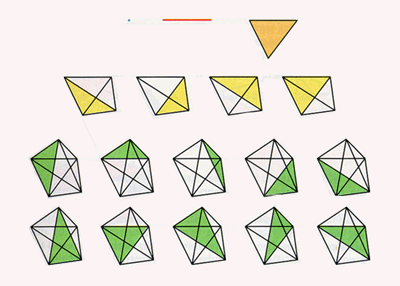The triangular faces of the simplexes in the first five dimensions.